## Multiplication Timed Test 0 12 PDF Details

Multiplication can be a difficult subject for students to learn. Thankfully, there are online tools that can help them practice and improve their skills. One of these tools is the multiplication timed test 0 12 form. This form provides students with a timed multiplication quiz that covers numbers 0 through 12. It also includes a score-tracking feature, so students can see how well they are doing over time. This tool is ideal for students who want to improve their multiplication skills quickly and easily.

Below, there are a number of particulars about multiplication timed test 0 12 PDF. It is suggested that you read this information before you start fiddling with the file.

Form NameMultiplication Timed Test 0 12
Form Length20 pages
Fillable?Yes
Fillable fields629
Avg. time to fill out32 min 42 sec
Other namesmultiplication quiz printable, multiplication facts 0 12 printable pdf, multiplication timed test pdf, multiplication timed test printable 0 12

## Form Preview ExampleMultiplication Facts 0-12 Worksheet A Item 4007-A

Minute Marker

NAME_____________________________

DATE _____________ SCORE ______/100

1 2 3

4

5

Multiplication Facts 0 - 12

Five minute timed drill with 100 problems.

4 6 7 0 2 7 5 2 10 9

× 4 × 3 × 4 × 0 × 2 × 1 × 3 × 1 × 7 × 1

8 12 11 10 3 11 5 3 12 10

× 0 × 6 × 5 × 8 × 1 × 9 × 2 × 3 × 4 × 1

10 12 10 9 11 4 10 12 7 4

× 10 × 0 × 2 × 7 × 8 × 3 × 5 × 9 × 5 × 1

11 7 6 4 12 10 6 8 10 6

× 10 × 0 × 5 × 0 × 8 × 6 × 2 × 8 × 3 × 6

12 9 5 11 9 3 11 7 2 8

× 12 × 8 × 0 × 3 × 6 × 2 × 7 × 2 × 0 × 4

11 4 10 12 7 10 9 12 9 7

× 11 × 2 × 4 × 3 × 3 × 5 × 2 × 5 × 3 × 6

12 11 10 7 1 10 9 6 8 6

× 11 × 0 × 9 × 7 × 0 × 0 × 4 × 4 × 1 × 0

11 6 12 11 11 9 8 5 9 8

× 4 × 1 × 1 × 6 × 12 × 5 × 5 × 1 × 9 × 6

 5 12 11 8 5 9 12 9 10 3 × 4 × 9 × 1 × 2 × 5 × 9 × 2 × 0 × 8 × 0 12 12 12 8 1 3 2 8 7 0 × 10 × 9 × 7 × 7 × 1 × 8 × 9 × 3 × 9 × 6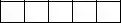Multiplication Facts 0-12 Worksheet A1 Item 4007-A1

Minute Marker

NAME_____________________________

DATE _____________ SCORE ______/100

1 2 3 4 5

Multiplication Facts 0 - 12

Five minute timed drill with 100 problems.

 4 × 4 =_____ 6 × 3 =_____ 7 × 4 =_____ 0 × 0 =_____ 2 × 2 =_____ 7 × 1 =_____ 5 × 3 =_____ 2 ×1 =_____ 10 × 7 =_____ 9 × 1 =_____ 8 × 0 =_____ 12 × 6 =_____ 11 × 5 =_____ 10 × 8 =_____ 3 × 1 =_____ 11 × 9 =_____ 5 × 2 =_____ 3 × 3 =_____ 12 × 4 =_____ 10 × 1 =_____ 10×10 =_____ 12 × 0 =_____ 10 × 2 =_____ 9 × 7 =_____ 11 × 8 =_____ 4 × 3 =_____ 10 × 5 =_____ 12 × 9 =_____ 7 × 5 =_____ 4 × 1 =_____ 11 –10 =_____ 7 × 0 =_____ 6 × 5 =_____ 4 × 0 =_____ 12 × 8 =_____ 10 × 6 =_____ 6 × 2 =_____ 8 × 8 =_____ 10 × 3 =_____ 6 × 6 =_____ 12×12 =_____ 9 × 8 =_____ 5 × 0 =_____ 11 × 3 =_____ 9 × 6 =_____ 3 × 2 =_____ 11 × 7 =_____ 7 × 2 =_____ 2 × 0 =_____ 8 × 4 =_____ 11×11 =_____ 4 × 2 =_____ 10 × 4 =_____ 12 × 3 =_____ 7 × 3 =_____ 10 × 5 =_____ 9 × 2 =_____ 12 × 5 =_____ 9 × 3 =_____ 7 × 6 =_____ 12×11 =_____ 11 × 0 =_____ 10 × 9 =_____ 7 × 7 =_____ 1 × 0 =_____ 10 × 0 =_____ 9 × 4 =_____ 6 × 4 =_____ 8 × 1 =_____ 6 × 0 =_____ 11 × 4 =_____ 6 × 1 =_____ 12 × 1 =_____ 11 × 6 =_____ 11×12 =_____ 9 × 5 =_____ 8 × 5 =_____ 5 × 1 =_____ 9 × 9 =_____ 8 × 6 =_____ 5 × 4 =_____ 12 × 9 =_____ 11 × 1 =_____ 8 × 2 =_____ 5 × 5 =_____ 9 × 9 =_____ 12 × 2 =_____ 9 × 0 =_____ 10 × 8 =_____ 3 × 0 =_____ 12×10 =_____ 12 × 9 =_____ 12 × 7 =_____ 8 × 7 =_____ 1 × 1 =_____ 3 × 8 =_____ 2 × 9 =_____ 8 × 3 =_____ 7 × 9 =_____ 0 × 6 =_____Multiplication Facts 0-12 Worksheet ─ Item 4007-─

Minute Marker

NAME_____________________________

DATE _____________ SCORE ______/100

1

2 3

4

5

Multiplication Facts 0 - 12

Five minute timed drill with 100 problems.

 11 8 10 5 7 12 1 9 11 4 × 1 × 5 × 9 × 0 × 4 × 8 × 0 × 5 × 6 × 2 7 10 0 9 6 9 12 8 10 12 × 6 × 5 × 0 × 3 × 3 × 1 × 11 × 8 × 7 × 0 12 7 3 10 11 12 9 11 2 6 × 9 × 3 × 2 × 0 × 10 × 6 × 9 × 9 × 1 × 5 9 11 12 6 5 12 2 9 11 12 × 4 × 8 × 5 × 1 × 4 × 3 × 0 × 2 × 7 × 4 9 10 5 4 1 8 3 11 12 8 × 8 × 6 × 3 × 8 × 1 × 6 × 3 × 4 × 10 × 0 12 8 7 10 4 11 9 8 6 4 × 1 × 3 × 5 × 4 × 1 × 3 × 6 × 2 × 6 × 3 3 12 6 10 11 12 5 11 7 8 × 9 × 7 × 4 × 3 × 5 × 12 × 2 × 0 × 2 × 4 11 5 4 6 10 8 2 10 7 3 × 11 × 9 × 0 × 8 × 2 × 7 × 2 × 2 × 1 × 0 12 10 9 10 7 8 10 3 4 4 × 2 × 1 × 7 × 8 × 0 × 9 × 10 × 1 × 7 × 4 6 5 3 8 7 5 11 3 2 12 × 0 × 5 × 7 × 1 × 7 × 6 × 9 × 9 × 5 × 6Multiplication Facts 0-12 Worksheet B1 Item 4007-B1

Minute Marker

NAME_____________________________

DATE _____________ SCORE ______/100

1

2 3 4 5

Multiplication Facts 0 - 12

Five minute timed drill with 100 problems.

 11 × 1 =_____ 8 × 5 =_____ 10 × 9 =_____ 5 × 0 =_____ 7 × 4 =_____ 12 × 8 =_____ 1 × 0 =_____ 9 × 5 =_____ 11 × 6 =_____ 4 × 2 =_____ 7 × 6 =_____ 10 × 5 =_____ 0 × 0 =_____ 9 × 3 =_____ 6 × 3 =_____ 9 × 1 =_____ 12×11 =_____ 8 × 8 =_____ 10 × 7 =_____ 12 × 0 =_____ 12 × 9 =_____ 7 × 3 =_____ 3 × 2 =_____ 10 × 0 =_____ 11×10 =_____ 12 × 6 =_____ 9 × 9 =_____ 11 × 9 =_____ 2 × 1 =_____ 6 × 5 =_____ 9 × 4 =_____ 11× 8 =_____ 12 × 5 =_____ 6 × 1 =_____ 5 × 4 =_____ 12 × 3 =_____ 2 × 0 =_____ 9 × 2 =_____ 11 × 7 =_____ 12 × 4 =_____ 9 × 8 =_____ 10 × 6 =_____ 5 × 3 =_____ 4 × 8 =_____ 1 × 1 =_____ 8 × 6 =_____ 3 × 3 =_____ 11 × 4 =_____ 12×10 =_____ 8 × 0 =_____ 12 × 1 =_____ 8 × 3 =_____ 7 × 5 =_____ 10 × 4 =_____ 4 × 1 =_____ 11× 3 =_____ 9 × 6 =_____ 8 × 2 =_____ 6 × 6 =_____ 4 × 3 =_____ 3 × 9 =_____ 12 × 7 =_____ 6 × 4 =_____ 10 × 3 =_____ 11 × 5 =_____ 12×12 =_____ 5 × 2 =_____ 11 × 0 =_____ 7 × 2 =_____ 8 × 4 =_____ 11×11 =_____ 5 × 9 =_____ 4 × 0 =_____ 6 × 8 =_____ 10 × 2 =_____ 8 × 7 =_____ 2 × 2 =_____ 10 × 2 =_____ 7 × 1 =_____ 3 × 0 =_____ 12 × 2 =_____ 10 × 1 =_____ 9 × 7 =_____ 10 × 8 =_____ 7 × 0 =_____ 8 × 9 =_____ 10×10 =_____ 3 × 1 =_____ 4 × 7 =_____ 4 × 4 =_____ 6 × 0 =_____ 5 × 5 =_____ 3 × 7 =_____ 8 × 1 =_____ 7 × 7 =_____ 5 × 6 =_____ 11 × 9 =_____ 3 × 9 =_____ 2 × 5 =_____ 12 × 6 =_____ Copyright ©2007 T. Smith Publishing. All rights reserved. www.tlsbooks.comMultiplication Facts 0-12 Worksheet C Item 4007-C

Minute Marker

NAME_____________________________

DATE _____________ SCORE ______/100

1

2 3

4

5

Multiplication Facts 0 -12

Five minute timed drill with 100 problems.

 11 2 12 5 10 9 7 10 11 4 × 7 × 0 × 4 × 2 × 8 × 6 × 2 × 3 × 9 × 1 12 8 9 10 3 11 12 0 6 8 × 2 × 5 × 9 × 0 × 1 × 6 × 9 × 0 × 3 × 2 7 11 9 6 10 12 7 3 9 5 × 4 × 3 × 4 × 0 × 7 × 8 × 6 × 2 × 5 × 0 9 4 10 12 1 12 6 5 8 11 × 0 × 2 × 5 × 7 × 0 × 5 × 4 × 1 × 0 × 4 11 8 5 10 11 12 7 3 8 9 × 2 × 7 × 4 × 6 × 10 × 11 × 0 × 3 × 6 × 7 12 9 3 10 12 11 5 11 2 6 × 1 × 2 × 6 × 9 × 3 × 1 × 3 × 0 × 1 × 6 3 12 8 5 10 2 7 6 4 8 × 0 × 6 × 1 × 5 × 2 × 6 × 5 × 1 × 0 × 4 12 6 4 9 10 9 11 7 12 7 × 0 × 2 × 3 × 1 × 10 × 8 × 5 × 1 ×10 × 7 11 6 2 11 10 12 7 2 3 8 × 11 × 5 × 2 × 8 × 1 × 12 × 3 × 8 × 5 × 3 10 9 4 5 7 2 9 3 4 2

×4 × 3 × 9 × 7 × 8 × 4 × 7 × 4 × 6 × 7

WWW.TLSBOOKS.COMMultiplication Facts 0-12 Worksheet C1 Item 4007-C1

Minute Marker

NAME_____________________________

DATE _____________ SCORE ______/100

1

2 3 4 5

Multiplication Facts 0 - 12

Five minute timed drill with 100 problems.

 11 × 7 =_____ 2 × 0 =_____ 12 × 4 =_____ 5 × 2 =_____ 10 × 8 =_____ 9 × 6 =_____ 7 × 2 =_____ 10 × 3 =_____ 11 × 9 =_____ 4 × 1 =_____ 12 × 2 =_____ 8 × 5 =_____ 9 × 9 =_____ 10 × 0 =_____ 3 × 1 =_____ 11 × 6 =_____ 12 × 9 =_____ 0 × 0 =_____ 6 × 3 =_____ 8 × 2 =_____ 7 × 4 =_____ 11 × 3 =_____ 9 × 4 =_____ 6 × 0 =_____ 10 × 7 =_____ 12 × 8 =_____ 7 × 6 =_____ 3 × 2 =_____ 9 × 5 =_____ 5 × 0 =_____ 9 × 0 =_____ 4 × 2 =_____ 10 × 5 =_____ 12 × 7 =_____ 1 × 0 =_____ 12 × 5 =_____ 6 × 4 =_____ 5 × 1 =_____ 8 × 0 =_____ 11 × 4 =_____ 11 × 2 =_____ 8 × 7 =_____ 5 × 4 =_____ 10 × 6 =_____ 11×10 =_____ 12×11 =_____ 7 × 0 =_____ 3 × 3 =_____ 8 × 6 =_____ 9 × 7 =_____ 12 × 1 =_____ 9 × 2 =_____ 3 × 6 =_____ 10 × 9 =_____ 12 × 3 =_____ 11 × 1 =_____ 5 × 3 =_____ 11 × 0 =_____ 2 × 1 =_____ 6 × 6 =_____ 3 × 0 =_____ 12 × 6 =_____ 8 × 1 =_____ 5 × 5 =_____ 10 × 2 =_____ 2 × 6 =_____ 7 × 5 =_____ 6 × 1 =_____ 4 × 0 =_____ 8 × 4 =_____ 12 × 0 =_____ 6 × 2 =_____ 4 × 3 =_____ 9 × 1 =_____ 10×10 =_____ 9 × 8 =_____ 11 × 5 =_____ 7 × 1 =_____ 12×10 =_____ 7 × 7 =_____ 11×11 =_____ 6 × 5 =_____ 2 × 2 =_____ 11× 8 =_____ 10 × 1 =_____ 12×12 =_____ 7 × 3 =_____ 2 × 8 =_____ 3 × 5 =_____ 8 × 3 =_____ 2 × 4 =_____ 9 × 3 =_____ 4 × 9 =_____ 5 × 7 =_____ 7 × 8 =_____ 6 × 4 =_____ 9 × 7 =_____ 3 × 4 =_____ 4 × 6 =_____ 2 × 7 =_____ Copyright ©2007 T. Smith Publishing. All rights reserved. www.tlsbooks.comMultiplication Facts 0-12 Worksheet D Item 4007-D

Minute Marker

NAME_____________________________

DATE _____________ SCORE ______/100

1 2 3

4 5

Multiplication Facts 0 - 12

Five minute timed drill with 100 problems.

 9 10 12 6 2 7 11 8 9 10 × 8 × 5 × 9 × 4 × 1 × 3 × 5 × 0 × 2 × 1 12 9 4 10 11 7 1 5 8 9 × 2 × 6 × 2 × 3 × 1 × 0 × 1 × 2 × 6 × 3 6 0 10 10 4 7 3 12 11 10 × 7 × 4 × 8 × 6 × 8 × 5 × 0 × 6 × 9 × 0 10 9 5 12 11 1 2 12 5 2 × 10 × 5 × 3 × 5 × 0 × 9 × 6 × 0 × 4 × 2 3 11 7 12 8 6 10 11 12 1 × 1 × 8 × 4 × 11 × 1 × 6 × 4 × 7 × 8 × 0 0 3 12 10 12 8 9 10 5 4 × 9 × 8 × 4 × 7 × 10 × 5 × 9 × 9 × 0 × 1 11 11 7 12 4 3 3 8 7 11 × 4 × 10 × 1 × 12 × 9 × 2 × 9 × 7 × 2 × 3 12 11 10 4 8 7 6 5 4 2 × 3 × 6 × 2 × 6 × 2 × 7 × 5 × 1 × 3 × 0 3 4 0 5 6 1 11 10 8 6 × 5 × 7 × 0 × 5 × 1 × 5 × 12 × 11 × 8 × 0 11 6 4 11 12 5 7 9 12 5 × 11 × 3 × 4 × 2 × 1 × 8 × 9 × 4 × 7 × 6Multiplication Facts 0-12 Worksheet D1 Item 4007-D1

Minute Marker

NAME_____________________________

DATE _____________ SCORE ______/100

1 2 3 4 5

Multiplication Facts 0 - 12

Five minute timed drill with 100 problems.

 9 × 8 =_____ 10 × 5 =_____ 12 × 9 =_____ 6 × 4 =_____ 2 × 1 =_____ 7 × 3 =_____ 11 × 5 =_____ 8 × 0 =_____ 9 × 2 =_____ 10 × 1 =_____ 12 × 2 =_____ 9 × 6 =_____ 4 × 2 =_____ 10 × 3 =_____ 11 × 1 =_____ 7 × 0 =_____ 1 × 1 =_____ 5 × 2 =_____ 8 × 6 =_____ 9 × 3 =_____ 6 × 7 =_____ 0 × 4 =_____ 10 × 8 =_____ 10 × 6 =_____ 4 × 8 =_____ 7 × 5 =_____ 3 × 0 =_____ 12 × 6 =_____ 11 × 9 =_____ 10 – 0 =_____ 10×10 =_____ 9 × 5 =_____ 5 × 3 =_____ 12 × 5 =_____ 11 × 0 =_____ 1 × 9 =_____ 2 × 6 =_____ 12 × 0 =_____ 5 × 4 =_____ 2 × 2 =_____ 3 × 1 =_____ 11 × 8 =_____ 7 × 4 =_____ 12×11 =_____ 8 × 1 =_____ 6 × 6 =_____ 10 × 4 =_____ 11 × 7 =_____ 12 × 8 =_____ 1 × 0 =_____ 0 × 9 =_____ 3 × 8 =_____ 12 × 4 =_____ 10 × 7 =_____ 12×10 =_____ 8 × 5 =_____ 9 × 9 =_____ 10 × 9 =_____ 5 × 0 =_____ 4 × 1 =_____ 11 × 4 =_____ 11×10 =_____ 7 × 1 =_____ 12×12 =_____ 4 × 9 =_____ 3 × 2 =_____ 3 × 9 =_____ 8 × 7 =_____ 7 × 2 =_____ 11 × 3 =_____ 12 × 3 =_____ 11 × 6 =_____ 10 × 2 =_____ 4 × 6 =_____ 8 × 2 =_____ 7 × 7 =_____ 6 × 5 =_____ 5 × 1 =_____ 4 × 3 =_____ 2 × 0 =_____ 3 × 5 =_____ 4 × 7 =_____ 0 × 0 =_____ 5 × 5 =_____ 6 × 1 =_____ 1 × 5 =_____ 11×12 =_____ 10×11 =_____ 8 × 8 =_____ 6 × 0 =_____ 11×11 =_____ 6 × 3 =_____ 4 × 4 =_____ 11 × 2 =_____ 12 × 1 =_____ 5 × 8 =_____ 7 × 9 =_____ 9 × 4 =_____ 12 × 7 =_____ 5 × 6 =_____Multiplication Facts 0-12 Worksheet E Item 4007-E

Minute Marker

NAME_____________________________

DATE _____________ SCORE ______/100

1 2 3

4

5

Multiplication Facts 0 - 12

Five minute timed drill with 100 problems.

 7 9 11 2 5 10 12 8 11 12 × 0 × 2 × 4 × 1 × 0 × 6 × 3 × 5 × 7 × 9 5 12 9 11 10 4 8 12 8 9 × 5 × 7 × 6 × 5 × 0 × 1 × 7 × 5 × 0 × 3 11 8 3 6 12 11 5 2 10 12 × 9 × 6 × 2 × 4 × 1 × 2 × 3 × 0 × 4 ×11 3 12 10 6 12 7 0 4 9 6 × 6 × 4 × 5 × 7 × 10 × 3 × 0 × 2 × 0 × 5 11 4 10 8 5 12 12 10 7 4 × 8 × 3 × 1 × 4 × 1 × 6 × 8 × 8 × 5 × 0 4 7 9 11 6 10 3 6 5 8 × 4 × 2 × 5 × 6 × 2 × 7 × 4 × 0 × 4 × 3 11 1 10 3 9 12 9 3 8 11 × 3 × 1 × 9 × 9 × 7 × 0 × 4 × 1 × 2 × 1 12 9 6 5 11 10 9 8 11 8 × 12 × 8 × 1 × 2 × 0 × 3 × 9 × 1 × 10 × 8 1 7 5 4 1 3 5 10 9 10 × 6 × 7 × 7 × 6 × 7 × 0 × 9 × 2 × 1 × 10 2 6 2 4 6 8 11 3 4 3 × 2 × 9 × 5 × 7 × 6 × 9 × 11 × 3 × 7 × 4Multiplication Facts 0-12 Worksheet E1

Item 4007-1 ̀

Minute Marker

NAME_____________________________

DATE _____________ SCORE ______/100

1 2 3 4 5

Multiplication Facts 0 - 12

Five minute timed drill with 100 problems.

 7 × 0 =_____ 9 × 2 =_____ 11 × 4 =_____ 2 × 1 =_____ 5 × 0 =_____ 10 × 6 =_____ 12 × 3 =_____ 8 × 5 =_____ 11 × 7 =_____ 12 × 9 =_____ 5 × 5 =_____ 12 × 7 =_____ 9 × 6 =_____ 11 × 5 =_____ 10 × 0 =_____ 4 × 1 =_____ 8 × 7 =_____ 12 × 5 =_____ 8 × 0 =_____ 9 × 3 =_____ 11 × 9 =_____ 8 × 6 =_____ 3 × 2 =_____ 6 × 4 =_____ 12 × 1 =_____ 11 × 2 =_____ 5 × 3 =_____ 2 × 0 =_____ 10 × 4 =_____ 12×11 =_____ 3 × 6 =_____ 12 × 4 =_____ 10 × 5 =_____ 6 × 7 =_____ 12×10 =_____ 7 × 3 =_____ 0 × 0 =_____ 4 × 2 =_____ 9 × 0 =_____ 6 × 5 =_____ 11 × 8 =_____ 4 × 3 =_____ 10 × 1 =_____ 8 × 4 =_____ 5 × 1 =_____ 12 × 6 =_____ 12 × 8 =_____ 10 × 8 =_____ 7 × 5 =_____ 4 × 0 =_____ 4 × 4 =_____ 7 × 2 =_____ 9 × 5 =_____ 11 × 6 =_____ 6 × 2 =_____ 10 × 7 =_____ 3 × 4 =_____ 6 × 0 =_____ 5 × 4 =_____ 8 × 3 =_____ 11 × 3 =_____ 1 × 1 =_____ 10 × 9 =_____ 3 × 9 =_____ 9 × 7 =_____ 12 × 0 =_____ 9 × 4 =_____ 3 × 1 =_____ 8 × 2 =_____ 11 × 1 =_____ 12×12 =_____ 9 × 8 =_____ 6 × 1 =_____ 5 × 2 =_____ 11 × 0 =_____ 10 × 3 =_____ 9 × 9 =_____ 8 × 1 =_____ 11×10 =_____ 8 × 8 =_____ 1 × 6 =_____ 7 × 7 =_____ 5 × 7 =_____ 4 × 6 =_____ 1 × 7 =_____ 3 × 0 =_____ 5 × 9 =_____ 10 × 2 =_____ 9 × 1 =_____ 10×10 =_____ 2 × 2 =_____ 6 × 9 =_____ 2 × 5 =_____ 7 × 4 =_____ 6 × 6 =_____ 8 × 9 =_____ 11 ×11=_____ 3 × 3 =_____ 4 × 7 =_____ 3 × 4 =_____

## How to Edit Multiplication Timed Test 0 12 Online for Free

The PDF editor which you'll use was designed by our main developers. It is possible to fill in the multiplication quiz printable document easily and effortlessly applying our app. Just keep up with the guide to get going.

Step 1: To start out, hit the orange button "Get Form Now".

Step 2: You're now on the file editing page. You can edit, add content, highlight certain words or phrases, put crosses or checks, and include images.

If you want to complete the multiplication quiz printable PDF, provide the details for all of the sections: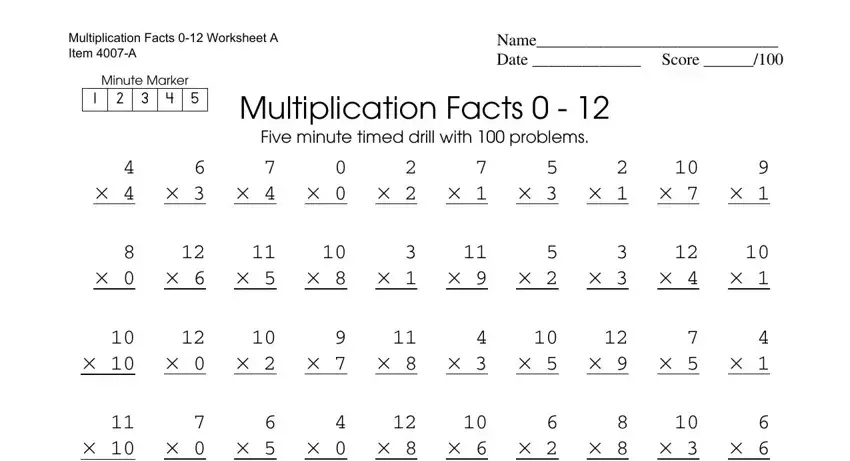Fill in the areas with any information that is required by the program.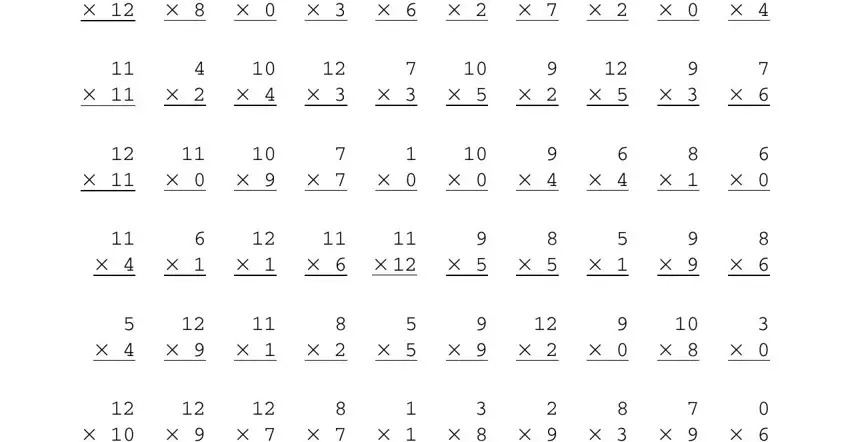In the field referring to www, tls, books, com you should jot down some essential data.The Multiplication, Facts, Worksheet, A, Item, A Name, Date, Score Minute, Marker Multiplication, Facts and Five, minute, timed, drill, with, problems box will be your place to include the rights and obligations of both sides.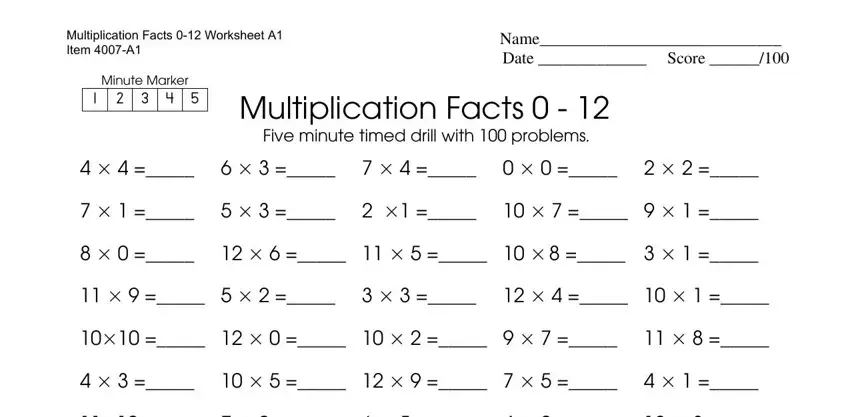Check the fields and then fill them out.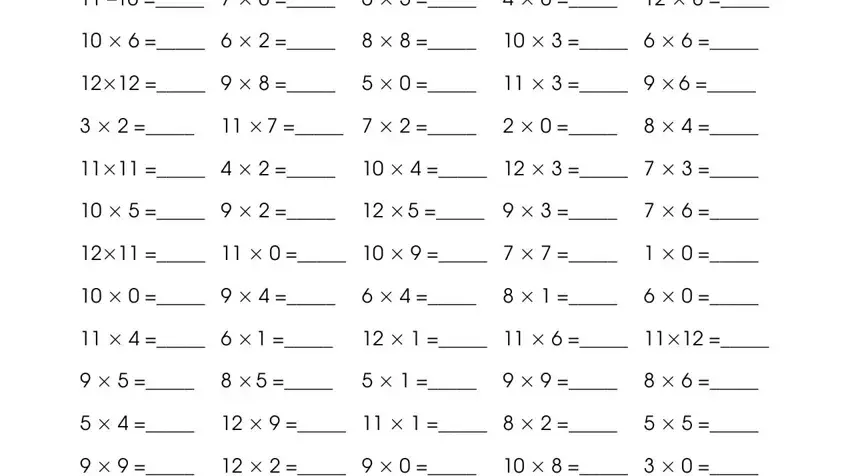Step 3: Click the "Done" button. Now it's easy to export your PDF document to your device. In addition, you can easily send it via email.

Step 4: Generate a minimum of several copies of your document to refrain from any sort of possible troubles.